# VBA Data Types – Numeric And Non-Numeric Data Types In VBA

This tutorial explains various numeric and non-numeric data types available in VBA. We will also learn about Data Type Conversions:

We come across many types of data every day like name, date, bills, price of a commodity, etc. All these data belong to a certain type and their values cannot defer from the type attached to it. Similarly, VBA has many types of data that are used to perform the required action.

In this tutorial, we will learn the different types of data used in VBA and see how those will help in organizing our program. We will also look into converting one data type to another data type.

=> Check ALL VBA Tutorials Here

## Categories Of Data Types

Data type tells the computer the type of data that needs to be stored by using a variable. Data types are divided into 2 categories i.e. Numeric and Non-Numeric Data Types

### Numeric Data Types

These types are used to perform mathematical operations like Addition, Subtraction, etc. Examples, are percentage calculation, share price, fees, bills, age, etc.

In VBA there are 7 types of numeric data types as mentioned below.

Numeric Data Type
1Byte
2Integer
3Long
4Single
5Double
6Currency
7Decimal

Let’s have a brief look at all the numeric data types.

#### #1) Byte Data Type

This data type requires just one byte of memory. Variables with data type Byte can store values from 0 to 255. The default byte value is 0. Negative values and values greater than 255 are not allowed. In case you attempt to assign invalid values, an Overflow error will be returned.

Syntax: Dim Vname As Byte

Here Vname is a variable name and Byte is the data type of the variable.

Example:

```Sub ByteDTtest()
Dim vname1 As Byte
vname1 = 10
MsgBox "Value of vname1 is : “ &vname1
End Sub```

The output is given below#### #2) Integer Data Type

These data types are used to store the whole integer value. This occupies 2 bytes of memory. An integer is one of the frequently used data types. They accept both positive values, negative values, and zero as well. They have a range between -32,768 to 32,767.

Syntax: Dim vname As Integer

Example:

```Sub IntDTtest()
Dim vname1, vname2 As Integer
vname1 = 10
vname2 = -20
MsgBox "Value of Integer variable is " & vname1 & " and " & vname2
End Sub```

The output is given below#### #3) Long Data Type

This is an alternative to an integer data type which also stores a whole integer value. However, it occupies more memory than an integer variable which is 4 bytes. It has a range of values from -2,147,483,648 to 2,147,483,648

Syntax: Dim vname As Long

```Sub IntDTtest()
Dim vname1, vname2 As Integer
vname1 = 10
vname2 = -20
MsgBox "Value of Integer variable is " & vname1 & " and " & vname2
End Sub```

If you execute the above code with an integer variable, you will get an overflow error because there are 1048576 rows in Excel and Integer data type only supports 32,767. As shown below when the data type is long, an appropriate result is shown.But when the data type is an integer, then an overflow error is thrown.#### #4) Decimal Data Type

This is an exact numeric data type that is used for its precision. That is the total number of digits and the number of digits to the right of the decimal point called a scaling factor.

In VBA, numbers are scaled by a power of 10. It’s appropriate to use these types while manipulating large numbers that need a precise value. This occupies 14 bytes in memory.

But variables cannot be directly declared as a decimal data type. In order to use Decimal, you must use the CDec conversion function. You need to use a Variant data type.

This data type contains the below range of values.

+/-79,228,162,514,264,337,593,543,950,335 with no decimal point
+/-7.9228162514264337593543950335 with 28 decimal places to the right of the decimal.
The smallest non-zero value accepted is +/-0.0000000000000000000000000001.

Syntax: Dim vname As variant

```Sub DecimalDataTypeTest()
Dim DecValue As Variant
DecValue = CDec(1000.4560323034)
MsgBox "Data type of Decvalue variable is : " & TypeName(DecValue) & " value " & DecValue
End Sub```

Note: The typeName function will give the datatype name

Output:#### #5) Single Data Type

This data type is used to store numbers with a single-precision floating-point. It occupies 4 bytes of memory. The default value is 0 and stores decimal values. You can use the Exclamation mark (!) while declaring a variable as shown in the syntax below.

The accepted range of values are:

3.402823E38 to -1.401298E-45 for negative values
1.401298E-45 to 3.402823E38 for positive values.

Syntax

Dim VariableName as Single
or
Dim VariableName!

```Sub SingleDataTypeTest()
Dim SingleVal1 As Single
Dim SingleVal2!
SingleVal1 = 123
SingleVal2 = 333.44
MsgBox ("Data type of SingleVal1 is " & TypeName(SingleVal1) & " with value " & SingleVal1 & "
and datatype of SingleVal2 is " & TypeName(SingleVal2) & " with value " & SingleVal2)
End Sub```

###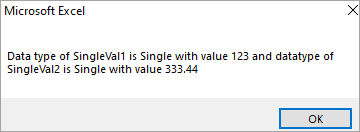#### #6) Double Data Type

The Double VBA data can be used to hold both integers and fractions. Double is used to store numbers with a Double-precision floating-point. It occupies 8 bytes of memory and has a range of values.

-1.79769313486231E308 to -4.94065645841247E-324 for negative values
4.94065645841247E-324 to 1.79769313486232E308 for positive values

Like the Single data type, double can also be declared using a symbol which is Hash (#) as shown below.

Syntax

Dim VariableName as Double
or
Dim VariableName#

```Sub DoubleDataTypeTest()
Dim douValue As Double
Dim douVal1#
douValue = 100.21
douVal1 = 333.44
MsgBox ("Data type of douValue is " & TypeName(douValue) & " with value "
& douValue & " and datatype of douVal1 is " & TypeName(douVal1) & " with value " & douVal1)
End Sub```

###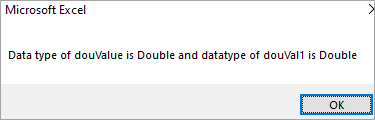#### #7) Currency Data Type

This data type can take up to 8 bytes of storage size. This type of data gives exact value, unlike single and double data types we discussed already are rounded. These are useful for monetary calculations.

The currency data type can store both positive and negative values. These can store 15 digits to the left of the decimal and 4 digits to the right.

Allowed range is -922,337,203,685,477.5808 to 922,337,203,685,477.5807. You can use @ to declare Currency data types.

Syntax

Dim VariableName as Currency
or
Dim VariableName@

```Sub CurrencyDataTypeTest()
Dim val1 As Currency
Dim Val2@
val1 = 123
Val2 = 333.44
MsgBox ("Data type of Val1 is " & TypeName(val1) &” with value “ &val1 & " and
datatype of Val2 is " & TypeName(Val2) & “ with value “ &val2)
End Sub```

Output:Note: If the value of the variable exceeds the provided range for a particular data type, then an overflow error will be thrown.

Consider the simple example of the byte data type exceeding its range. The same error will be displayed if you enter negative values for the byte data type.### Non-Numeric Data Types

These are data that can’t be manipulated by arithmetic operators. They comprise of Text, String, Date, etc. Given below are the non-numeric data types supported in VBA.

Non-Numeric Data Type
1String(Fixed Length)
2String(Variable Length)
3Date
4Boolean
5Object
6Variant(Numbers)
7Variant(Characters)

#### #1) Boolean Data Type

This data type requires 2 bytes of memory and can store only 2 values i.e. TRUE or FALSE. In other words, the Boolean variable can only get values of either TRUE or FALSE, alternatively 1 or 0 respectively. The default value of a Boolean variable is False.

Syntax – Dim Vname As Boolean

Example:

```Sub BooleanDataTypeTest()
Dim bval1 As Boolean
MsgBox ("datatype of variable bval1 is :" & TypeName(bval1) & " default value of boolean varible is " & bval1)
End Sub```#### #2) Date Data Type

This data type is used to represent the date and time. It has date range values from Jan 1, 0100 to December 31, 9999, and time values from 0:00:00 to 23:59:59 and occupies 8 bytes of storage size.

Syntax: Dim vname As Date

```Sub DateDataTypeTest()
Dim datetime As Date
datetime = Now
MsgBox "Current date and time is " & datetime
End Sub```

Note: Now function gives the current date and time#### #3) String Data Type

This data type is used to store string values. The string is defined as a sequence of characters. So you can use String data type to store text and can be used to store numbers, special characters, s, and event spaces. A string value should be embedded in a double quotation mark “ ”.

There are 2 types of String data types.

#1) Variable-length String: This type occupies 10 bytes of storage size plus the memory required for the string that is the string length. They have a range value from 0 to approximately 2 billion.

#2) Fixed-length String: It occupies the memory equal to the length of the string itself. It can range from 1 to approximately 65,400 characters

Syntax: Dim Vname As String

Example:

```Sub StringDataTypeTest()
Dim sVal1 As String
sVal1 = "Text1234#\$@ and Spaces:)"
MsgBox "I can accept anything " & sVal1
End Sub```

###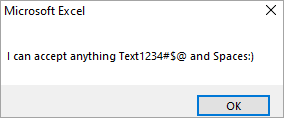#### #4) Object Data Type

The object will have a reference to an object of any type i.e. Object data type can point to any data type like string, double, integer, etc. Object variable won’t contain the value, it only points to the address in which the data is stored. It occupies 4 bytes of computer memory. The default value of an object is a null reference.

Syntax: Dim VName As Object

Example:

```Sub ObjectDataTypeTest()
Dim wsActiveSheet As Object
Set wsActiveSheet = ActiveSheet
wsActiveSheet.UsedRange.Clear
End Sub```

This will clear all the used cells in the current sheet.

#### #5) Variant Data Type

This is the universal data type of VBA, it can accept any kind of data numeric and non-numeric. Variant data type gives more flexibility while working with data. Variant data type uses more storage size than any other data type. If you don’t mention a datatype, VBA will treat that as a Variant variable.

There are 2 types of Variant data types

#1) Variant (numbers): This can contain any numeric value up to the range of Double. The Variant numbers occupy 16 bytes of storage size.

#2) Variant (characters): This can contain the same range as for variable-length String. The Variant characters occupy 22 bytes + string length (24 bytes on 64-bit systems)

Syntax

Dim VName As Variant
or
Dim VName

Example:

```Sub VariantDataTypeTest()
Dim EmpName As Variant
Dim DOB
Dim Salary As Variant

EmpName = "Jofn kim"
DOB = #10/3/2020#
Salary = 55000
MsgBox EmpName & " " & DOB & " " & Salary
End Sub```

### Data Type Conversion

Sometimes it becomes necessary to convert the data type of a variable to something specific in our use case.

Example: You retrieve a value from a cell, which is usually a string, and hence you need to convert it to a numeric data type before performing any arithmetic operation. To achieve this VBA has type conversion functions for all the supported data types.

#### #1) CBool

This function is used to convert an expression to a Boolean data type. If the expression returns zero then CBool will return False, for any nonzero value, CBool returns True.

```Sub CBooleanTest()
Dim val1 As Integer
Dim strval2 As String
val1 = 0
MsgBox CBool(val1)
val1 = 177
MsgBox CBool(val1)
strval2 = "A"
MsgBox CBool(strval2 = "B")
MsgBox CBool(strval2 <> "B")
End Sub```

The above code will return results as False, True, False, True.

You can try it yourself, draw an ActiveX control command button, Right-click -> View code and insert the above code. Click on the Command button and the result will be displayed. (Disable the design mode)

Output#### #2) CByte

This function is used to convert an expression to a Byte data type. Remember after conversion if the range exceeds the range allowed for Byte, then an Overflow error will be thrown.

```Sub cbyteTest()
Dim val1 As Double
Dim val2
val1 = 125.5678
val2 = CByte(val1)
MsgBox ("DataType is " & TypeName(val2) & " and value of val2 is " & val2)
End Sub```

Note: If you give the value as 255.56. Compiler will return an overflow error#### #3) CCur

This function will convert an expression to a Currency data type.

```Sub ccurTest()
Dim val1 As Integer
Dim val2
val1 = 5544
val2 = CCur(val1 / 5)
MsgBox ("DataType is " & TypeName(val2) & " and value of val2 is " & val2)
End Sub```#### #4) CDate

This function will convert a string to a Date. Suppose you retrieve the Date value as a String from an excel cell, then you need to convert it before performing any further action. You can use CDate.

```Sub CDateTest()
Dim val1, val2, val3 As String
Dim Res1, Res2, Res3
val1 = "12:21"
val2 = "23/09 12:12"
val3 = "Sep 23, 2020"
Res1 = CDate(val1)
Res2 = CDate(val2)
Res3 = CDate(val3)
MsgBox "Date of val1 is : " & Res1 & " Date of val2 is : " & Res2 & " Date of val3 is " & Res3
End Sub
```

##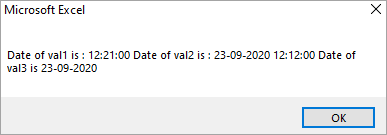#### #5) CDbl

CDbl function is used to convert an expression to a Double data type.

```Sub CDblTest()
Dim val1 As String
Dim val2 As Integer
Dim Res1
val1 = 10000.12345
val2 = 1222.222
Res1 = CDbl(val1)
Res2 = CDbl(val2)
MsgBox "Double value after converting String : " & Res1 & " Double value after converting Integer : " & Res2

End Sub```

##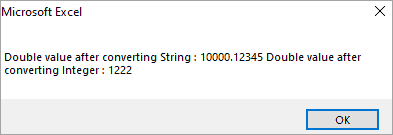#### #6) CDec

This function will convert a numeric value to a Decimal.

```Sub CDecTest()
Dim Val1 As Currency
Dim Result
Val1 = 1234566.56366
Result = CDec(Val1) ‘Result is 1234566.5637
MsgBox "Decimal Data Type Value : " & Result
End Sub```

###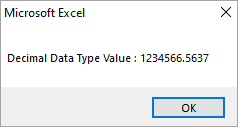#### #7) CInt

CInt function will convert a value to an Integer Data Type.

```Sub CintTest()
Dim Val1 As Double
Dim Result
Val1 = 2345.5678
Result = CInt(Val1) 'Result is 2346
MsgBox "Integer value of converting is : " & Result & " and datatype of Result is " & TypeName(Result)
End Sub```

##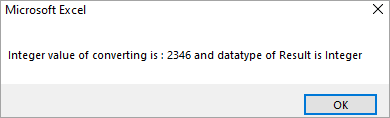#### #8) CLng

This function is used to convert a value to a Long Data Type

```Sub cLngTest()
Dim Val1, Val2 As Double
Dim Res1, Res2
Val1 = 45457.35
Val2 = 45457.65
Res1 = CLng(Val1)
Res2 = CLng(Val2)
MsgBox "Result after converting Res1 is " & Res1 & " and Res2 value is " & Res2
End Sub```

##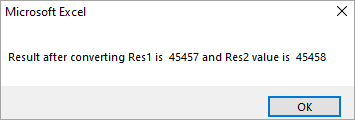#### #9) CSng

This function will convert a value to a Single Data Type

```Sub cSngTest()
Dim Val1, Val2 As Double
Dim Res1, Res2
Val1 = 75.3421115
Val2 = 75.3421555
Res1 = CSng(Val1) ' Result is 75.34211.
Res2 = CSng(Val2) ' Result is 75.34216.
MsgBox "Result after converting Res1 is " & Res1 & " and Res2 value is " & Res2
End Sub```

##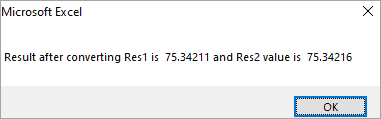#### #10) CStr

This function is used to convert a numeric value to a string data type.

```Sub CStrTest()
Dim Val1 As Double
Dim Result
Val1 = 123.123
Result = CStr(Val1) 'Result will be 123.123
MsgBox "Result of Val1 is " & Val1
End Sub```

##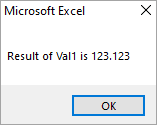#### #11) CVar

This function will convert an expression to a Variant data type.

```Sub CVarTest()
Dim Val1 As Integer
Dim Result
Val1 = 1234
Result = CVar(Val1 & 1.12) ' Result will be 12341.12
MsgBox "Result is " & Result
End Sub```

#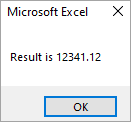Q #1) What is the default data type in VBA?

Answer: Variant is the default one. If you don’t define a data type of a variable then VBA treats it as a variant variable/object.

Q #2) What is a Type Mismatch error?

Answer: This error will be triggered when you declare a variable as one data type and assign an inappropriate value.

Example: Declare a variable as Integer and enter a text value.

Q #3) How do I fix an overflow error?

Answer: You need to refer to the range that particular data type is allowed and make sure that you enter a value within the allowed range.

Example: Byte allows only 0 to 255, if you enter any negative value or v value more than 255 then you encounter an overflow error.

Suggested reading =>> Error Handling in VBA

## Conclusion

In this tutorial, we have learned about VBA Data Types i.e. 7 numeric and 7 non-numeric data types. We also discussed how to convert a data type from one type to another with examples.

=> Check Here To See A-Z Of VBA Training Tutorials